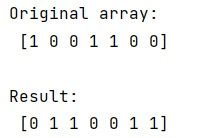# Flip zeros and ones in one-dimensional NumPy array

Flipping zeros and ones: In this tutorial, we will learn how to flip zeros and ones in one-dimensional NumPy array? By Pranit Sharma Last updated : May 12, 2023

Suppose that we are given a one-dimensional NumPy array that consists of zeros and ones. We need to find a quick way to just "flip" the values such that zeros become ones, and ones become zeros.

## How to flip zeros and ones in one-dimensional NumPy array?

To flip zeros and ones in a one-dimensional array, the quick and easiest way is to simply subtract each array element by 1 using 1-arr, another way is to define the data type of the array as bool and find the inverse of the array by using ~arr. It will flip all zeros and ones in a NumPy array. Consider the below code statement to achieve this:

```res = 1 - arr
```

Here, arr is an original one-dimensional NumPy array, and res will store the array containing the flipped zeros and ones.

Let us understand with the help of an example,

## Python program to flip zeros and ones in one-dimensional NumPy array

```# Import numpy
import numpy as np

# Creating an array
arr = np.array([1,0,0,1,1,0,0])

# Display original array
print("Original array:\n",arr,"\n")

# Flipping zeros and ones
res = 1-arr

# Display result
print("Result:\n",res,"\n")
```

### Output

```Original array:
[1 0 0 1 1 0 0]

Result:
[0 1 1 0 0 1 1]
```

### Output (Screenshot)